## Testing of DC Machine or Swinburne Test:

Testing of DC Machine or Swinburne Test – Machine efficiency, in general, has been discussed already. The approach here will be to apply the general principles for the specific case of dc machines. The power flow diagrams for the generating and motoring modes of a dc machine are shown in Fig. 7.65(a) and (b).

Certain symbols used in Figs 7.65(a) and (b) are redefined here.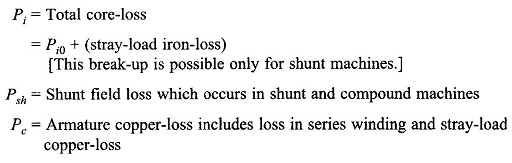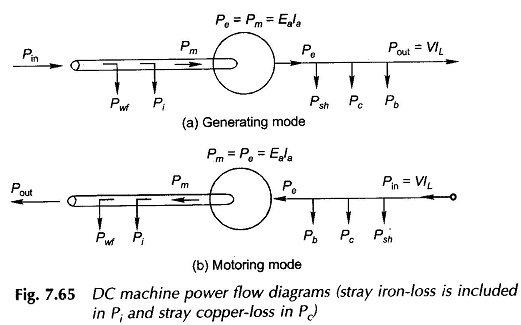The other symbols retain their usual meaning;

The expressions for efficiencies of generating and motoring machines with reference to Eqs (5.76a) and (5.78a) are given below: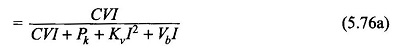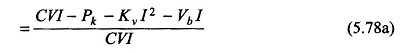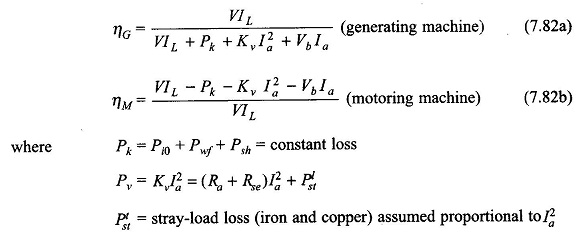It is known from Eq. (5.77) that the maximum efficiency occurs at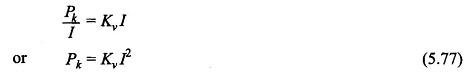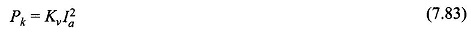There is a wide variety of non-loading tests that could be performed on dc machines. Swinbume’s test and Hopkinson’s test are the most important and actually conducted in practice on shunt motors. For obvious reasons the non-loading test cannot be conducted on a series motor.

Swinburne ‘s test: This is a no-load test and hence cannot be performed on a series motor. Figure 7.66 gives the connections for the Testing of DC Machine. The motor is run at no-load at rated speed. Field current is adjusted to a rated value for accurate determination of no-load loss (Pi0 + Pwf). The machine would run at higher than rated speed with a rated armature voltage. Therefore a series resistor in the armature circuit is employed to reduce voltage applied to the motor armature such that it runs at rated speed.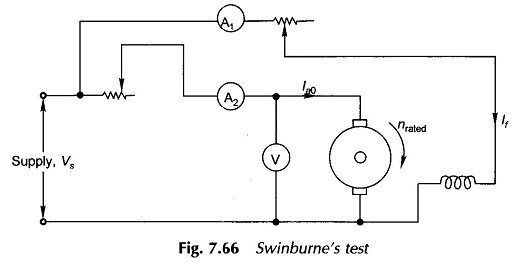Constant loss: In Fig. 7.66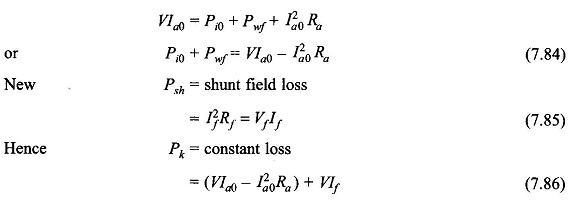### Variable loss:

The armature resistance (inclusive of brush contact drop assumed approximately linear) is measured by a dc test by passing a rated armature current from a battery supply. Then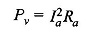The stray load-loss can be neglected or estimated as 1% of rated output at full load.

Efficiency can now be calculated at any load current on the following lines:

Motor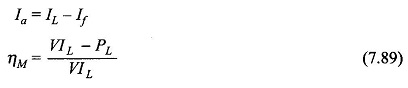Note: Since the resistances (Ra and Rf) are measured cold, temperature correction must be applied to these before using in efficiency calculations.

### Disadvantages in Testing of DC Machine:

• The stray-load loss cannot be determined by this test and hence efficiency is over-estimated. Correction can be applied by assuming the stray-load loss to be half the no-load loss.
• A steady temperature rise of the machine cannot be determined.
• The Testing of DC Machine does not indicate whether commutation would be satisfactory when the machine is loaded.
Scroll to Top